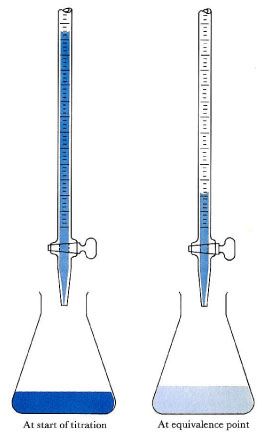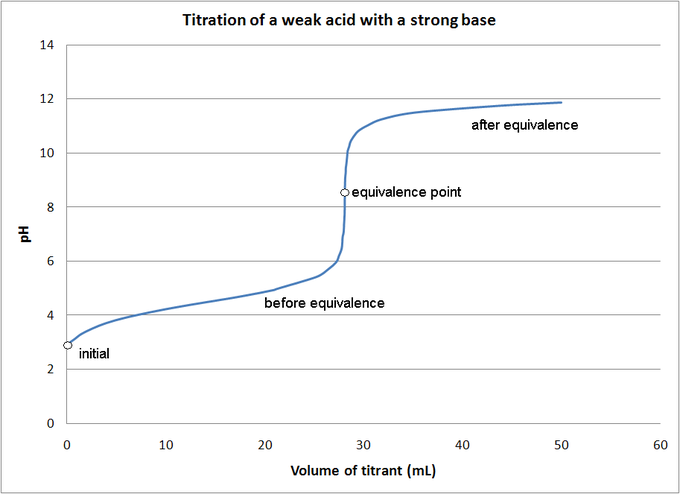Acid-Base Titrations

Learning Objective

• Compute the concentration of an unknown acid or base given its volume and the volume and concentration of the standardized titrant.

Key Points

• An acid-base titration is a quantitative analysis of acids and bases; through this process, an acid or base of known concentration neutralizes an acid or base of unknown concentration.
• The titration progress can be monitored by visual indicators, pH electrodes, or both.
• The reaction’s equivalence point is the point at which the titrant has exactly neutralized the acid or base in the unknown analyte; if you know the volume and concentration of the titrant at the equivalence point, you can calculate the concentration of a base or acid in the unknown solution.

Terms

• acid-base titrationdetermines the concentration of an acid or base by exactly neutralizing it with an acid or base of known concentration
• equivalence pointthe point at which an added titrant’s moles are stoichiometrically equal to the moles of acid/base in the sample; the smallest amount of titrant needed to fully neutralize or react with the analyte
• titrantthe standardized (known) solution (either an acid or a base) that is added during titration
• analytethe unknown solution whose concentration is being determined in the titration

Setting up an Acid-Base Titration

An acid-base titration is an experimental procedure used to determined the unknown concentration of an acid or base by precisely neutralizing it with an acid or base of known concentration. This lets us quantitatively analyze the concentration of the unknown solution. Acid-base titrations can also be used to quantify the purity of chemicals.Acid-base titrationThe solution in the flask contains an unknown number of equivalents of base (or acid). The burette is calibrated to show volume to the nearest 0.001 cm3. It is filled with a solution of strong acid (or base) of known concentration. Small increments are added from the burette until, at the end point, one drop changes the indicator color permanently. (An indication of the approaching equivalence point is the appearance, and disappearance after stirring, of the color that the indicator assumes beyond neutralization.) At the equivalence point, the total amount of acid (or base) is recorded from the burette readings. The number of equivalents of acid and base must be equal at the equivalence point.

Alkalimetry, or alkimetry, is the specialized analytic use of acid-base titration to determine the concentration of a basic (alkaline) substance; acidimetry, or acidometry, is the same concept applied to an acidic substance.

Materials for a Titration Procedure

• burette
• white tile (used to see a color change in the solution)
• pipette
• pH indicator (the type depends on the reactants)
• titrant (a standard solution of known concentration; a common example is aqueous sodium carbonate)
• analyte, or titrand (the solution of unknown concentration)

Equivalence Point Indicators

Before you begin the titration, you must choose a suitable pH indicator, preferably one that will experience a color change (known as the “end point”) close to the reaction’s equivalence point; this is the point at which equivalent amounts of the reactants and products have reacted. Below are some common equivalence point indicators:

• strong acid-strong base titration: phenolphthalein indicator
• weak acid-weak base titration: bromthymol blue indicator
• strong acid-weak base titration: methyl orange indicator the base is off the scale (e.g., pH > 13.5) and the acid has pH > 5.5: alizarine yellow indicator
• the base is off the scale (e.g., pH > 13.5) and the acid has pH > 5.5: alizarine yellow indicator
• the base is off the scale (e.g., pH > 13.5) and the acid has pH > 5.5: alizarine yellow indicator
• the acid is off the scale (e.g., pH < 0.5) and the base has pH < 8.5: thymol blue indicator

Estimating the Equivalence Point’s pH

The resulting solution at the equivalence point will have a pH dependent on the acid and base’s relative strengths. You can estimate the equivalence point’s pH using the following rules:

• A strong acid will react with a weak base to form an acidic (pH < 7) solution.
• A strong acid will react with a strong base to form a neutral (pH = 7) solution.
• A weak acid will react with a strong base to form a basic (pH > 7) solution.

When a weak acid reacts with a weak base, the equivalence point solution will be basic if the base is stronger and acidic if the acid is stronger; if both are of equal strength, then the equivalence pH will be neutral. Weak acids are not often titrated against weak bases, however, because the color change is brief and therefore very difficult to observe.

You can determine the pH of a weak acid solution being titrated with a strong base solution at various points; these fall into four different categories: (1) initial pH; (2) pH before the equivalence point; (3) pH at the equivalence point; and (4) pH after the equivalence point.Titration of a weak acid by a strong baseThe pH of a weak acid solution being titrated with a strong base solution can be found at each indicated point.

Titration Procedure

1. Rinse the burette with the standard solution, the pipette with the unknown solution, and the conical flask with distilled water.
2. Place an accurately measured volume of the analyte into the Erlenmeyer flask using the pipette, along with a few drops of indicator. Place the standardized solution into the burette, and indicate its initial volume in a lab notebook. At this stage, we want a rough estimate of the amount of known solution necessary to neutralize the unknown solution. Let the solution out of the burette until the indicator changes color, and record the value on the burette. This is the first titration and it is not very precise; it should be excluded from any calculations.
3. Perform at least three more titrations, this time more accurately, taking into account where the end point will roughly occur. Record the initial and final readings on the burette, prior to starting the titration and at the end point, respectively. (Subtracting the initial volume from the final volume will yield the amount of titrant used to reach the endpoint.)
4. The end point is reached when the indicator permanently changes color.